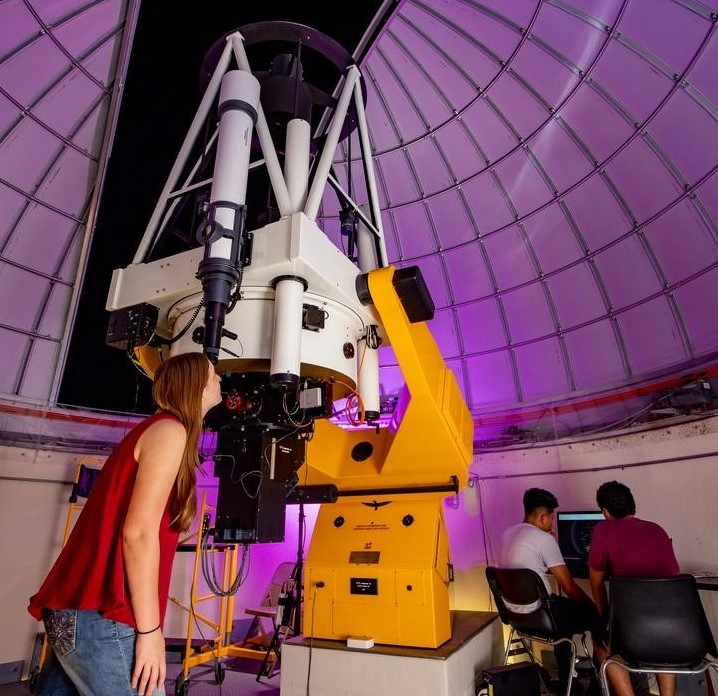## Aerospace, Physics, and Space Science Faculty Publications

Article

#### Publication Title

Physics Letters B

#### Abstract

Results are presented from a search for supersymmetric particles in scenarios with small mass splittings. The data sample corresponds to 19.7 fb-1 of proton-proton collisions recorded by the CMS experiment at s=8 TeV. The search targets top squark (t~) pair production in scenarios with mass differences δm=m(t~)-m(χ~10) below the W-boson mass and with top-squark decays in the four-body mode (t~→bℓνχ~10), where the neutralino (χ~10) is assumed to be the lightest supersymmetric particle (LSP). The signature includes a high transverse momentum (pT) jet associated with initial-state radiation, one or two low-pT leptons, and significant missing transverse energy. The event yields observed in data are consistent with the expected background contributions from standard model processes. Limits are set on the cross section for top squark pair production as a function of the t~ and LSP masses. Assuming a 100% branching fraction for the four-body decay mode, top-squark masses below 316 GeV are excluded for δm=25 GeV at 95% CL. The dilepton data are also interpreted under the assumption of chargino-neutralino production, with subsequent decays to sleptons or sneutrinos. Assuming a difference between the common χ~1+/χ~20 mass and the LSP mass of 20 GeV and a τ-enriched decay scenario, masses in the range m(χ~1+)<307 GeV are excluded at 95% CL.

#### DOI

10.1016/j.physletb.2016.05.033

2016

COinS